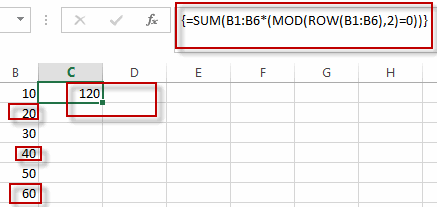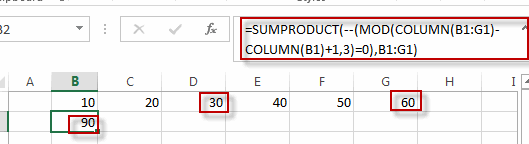# Sum Every Nth Row or Column

This post will guide you how to sum every nth row or column with a formula in Excel. How do I sum every nth value in a same row or column in Excel. How to sum every nth cell in a column or in a row with an Excel Formula. How to sum every second, third, or nth row or column in a range in Excel.

## Sum Every Nth Row

If you want to sum every nth rows in Excel, you can create an Excel Array formula based on the SUM function, the MOD function and the ROW function.
For example, you want to sum every 2 rows in the range B1:B6, you can write down this formula to achieve the result.

`=SUM(B1:B6*(MOD(ROW(B1:B6),2)=0))`

You need to type this formula into a blank cell, and press Ctrl +Shift +Enter shortcuts to change the formula as Array formula.You can change the number 2 as you need to sum every nth row in a specific range of cells in Excel.

## Sum Every Nth Column

If you want to sum every nth column in a column, you can create a formula based on the SUMPRODUCT function, the MOD function, and the Column function.

For example, you want to sum every 3rd column in range B1:G1, you can use the following formula to achieve the result.

`=SUMPRODUCT(--(MOD(COLUMN(B1:G1)-COLUMN(B1)+1,3)=0),B1:G1)`### Related Functions

• Excel SUMPRODUCT function
The Excel SUMPRODUCT function multiplies corresponding components in the given one or more arrays or ranges, and returns the sum of those products.The syntax of the SUMPRODUCT function is as below:= SUMPRODUCT (array1,[array2],…)…
• Excel SUM function
The Excel SUM function will adds all numbers in a range of cells and returns the sum of these values. You can add individual values, cell references or ranges in excel.The syntax of the SUM function is as below:= SUM(number1,[number2],…)…
• Excel MOD function
he Excel MOD function returns the remainder of two numbers after division. So you can use the MOD function to get the remainder after a number is divided by a divisor in Excel. The syntax of the MOD function is as below:=MOD (number, divisor)….
• Excel COLUMN function
The Excel COLUMN function returns the first column number of the given cell reference.The syntax of the COLUMN function is as below:=COLUMN ([reference])….
• Excel ROW function
The Excel ROW function returns the row number of a cell reference.The ROW function is a build-in function in Microsoft Excel and it is categorized as a Lookup and Reference Function.The syntax of the ROW function is as below:= ROW ([reference])….